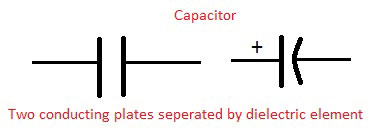# Capacitor

The capacitor is an electrical component used to store charge. A capacitor consists of two conducting plates separated by a dielectric. Capacitor has a properly to block d.c current and pass a.c. current. A passive component which has the ability to charge or store energy is called as capacitor. A capacitor was therefore historically first known as an electric condenser. The effect of a capacitor is called as capacitance. It is composed of two parallel conducting plates separated by non-conducting region that is called dielectric, such as vacuum, ceramic, air, aluminum, etc. Capacitance is defined as the ratio of the electric charge on each conductor to the potential difference between them. The unit of capacitance is the Farad (F) which is defined as coulomb per volt. The capacitance of a capacitor is proportional to the surface area of the plates (conductors) and inversely related to the gap between them. Capacitors are widely used in electronic circuits for blocking direct current while allowing alternating current to pass.Classification of capacitors: